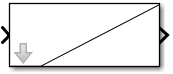# plot

Draw graph connecting series of points on masked subsystem icon

## Syntax

```plot(`Y`)
plot(`X1`,`Y1`,`X`2,`Y2`,...)

```

## Description

`plot(Y)` plots, for a vector `Y`, each element against its index. If `Y` is a matrix, it plots each column of the matrix as though it were a vector.

`plot(X1,Y1,X2,Y2,...)` plots the vectors `Y1` against `X1`, `Y2` against `X2`, and so on. Vector pairs must be the same length and the list must consist of an even number of vectors.

Plot commands can include `NaN` and `inf` values. When Simulink® software encounters `NaN`s or `inf`s, it stops drawing, and then begins redrawing at the next numbers that are not `NaN` or `inf`. The appearance of the plot on the icon depends on the units defined by the Icon units option in the Mask Editor.

Simulink software displays three question marks (`? ? ?`) in the block icon and issues warnings in these situations:

• When you have not defined values for the parameters used in the drawing commands (for example, when you first create the mask, but have not yet entered values in the Mask Settings dialog box)

• When you enter a masked block parameter or drawing command incorrectly

The `plot` command in masking supports a subset of the syntax of the MATLAB `plot` command. The `plot` command in masking supports all numeric data types and accepts only numbers as input.

## Examples

The command

```plot([0 1 5], [0 0 4]) ```

generates the plot that appears on the icon for the Ramp block, in the Sources library.